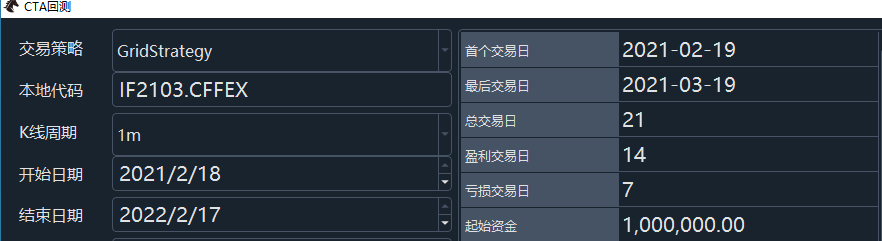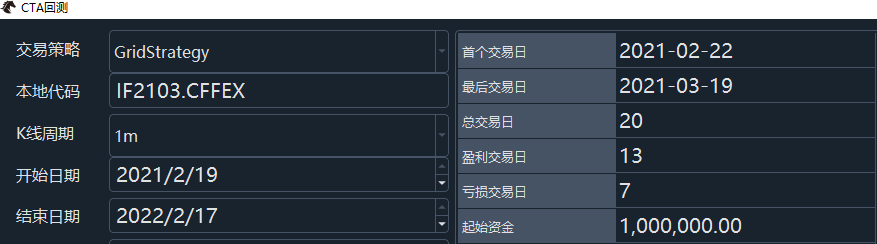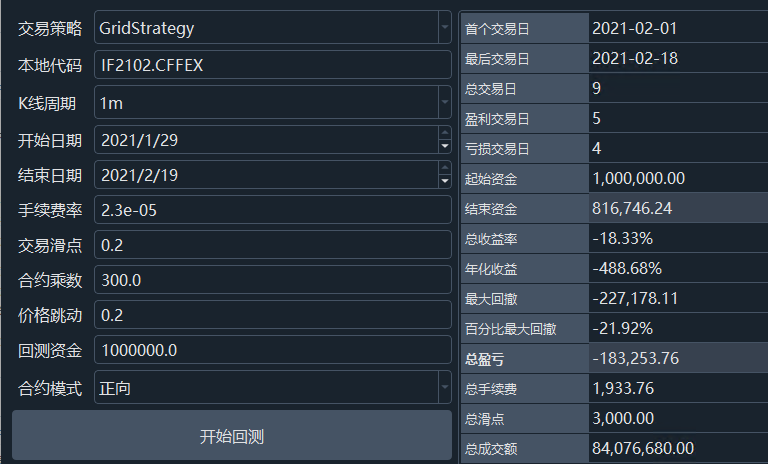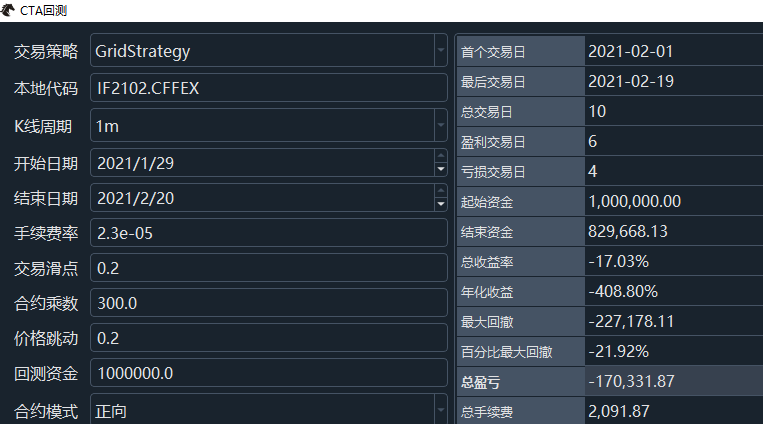VeighNa量化社区from vnpy_ctastrategy import (
CtaTemplate,
StopOrder,
TickData,
BarData,
OrderData,
BarGenerator,
ArrayManager,
)
import math

class GridStrategy(CtaTemplate):
""""""

``````author = "Fighter"

#定义参数

initial_price = 1.0
step_price = 1.0
step_volume = 1.0
max_pos = 4

vt_orderid = ""
pos = 0
parameters = ["initial_price", "step_price", "step_volume", "max_pos"]

def __init__(self, cta_engine, strategy_name, vt_symbol, setting):
""""""
super().__init__(cta_engine, strategy_name, vt_symbol, setting)
self.bg = BarGenerator(self.on_bar)
self.am = ArrayManager()

def on_init(self):
"""
Callback when strategy is inited.
"""
self.write_log("策略初始化")
self.pos = 0

def on_start(self):
"""
Callback when strategy is started.
"""
self.write_log("策略启动")
self.put_event()

def on_stop(self):
"""
Callback when strategy is stopped.
"""
self.write_log("策略停止")
self.put_event()

def on_tick(self, tick: TickData):
"""
Callback of new tick data update.
"""
self.bg.update_tick(tick)

def on_bar(self, bar: BarData):
"""
Callback of new bar data update.
"""

if abs(bar.close_price - self.trade_price)/self.step_price * 0.8 < 1:
return

if self.initial_price > bar.close_price:
target_trade_distance = (self.initial_price - bar.close_price) / self.step_price

self.initial_price = self.initial_price - self.step_price * target_trade_position / 2

elif self.initial_price < bar.close_price:

target_trade_distance = (bar.close_price - self.initial_price) / self.step_price

self.initial_price = self.initial_price - self.step_price * target_trade_position / 2

def on_order(self, order: OrderData):
"""
Callback of new order data update.
"""
pass

"""
Callback of new trade data update.
"""
self.put_event()

def on_stop_order(self, stop_order: StopOrder):
"""
Callback of stop order update.
"""
pass``````

self,
days: int,
interval: Interval = Interval.MINUTE,
callback: Callable = None,
use_database: bool = False
):

https://www.vnpy.com/docs/cn/cta_strategy.html

xiaohe wrote:

https://www.vnpy.com/docs/cn/cta_strategy.html

xiaohe wrote: